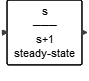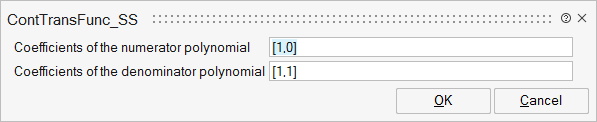# ContTransFunc_SS

This block implements a SIMO (continuous-time, single-input, multi-output) linear system that is defined by the linear system's transfer function. The transfer function, specified by the coefficients of the function's numerator and denominator, must be proper, meaning that the degree of the numerator must not exceed that of the denominator. The initial state is computed such that the system is initially in steady-state.## Description

This block implements a SIMO (continuous-time, single-input, multi-output) linear system that is defined by the linear system's transfer function. The transfer function, specified by the coefficients of the function's numerator and denominator, must be proper, meaning that the degree of the numerator must not exceed that of the denominator. The initial state is computed such that the system is initially in steady-state.

## ParametersNameLabelDescriptionData TypeValid Values

num

Coefficients of the numerator polynomial

A mxn matrix, m is the number of output, n is the order of the numerator polynomial. This must be a polynomial in s domain.

Matrix

den

Coefficients of the denominator polynomial

Defines the denominator of the transfer function.

Vector

## Ports

NameTypeDescriptionIO TypeNumber

Port 1

explicit

output

1

Port 2

explicit

input

1

NameValueDescription

always active

yes

direct-feedthrough

no

no, unless the order of numerator is equal to the order of denominator.

zero-crossing

no

mode

no

continuous-time state

yes

discrete-time state

no